# Balbharati solutions for Mathematics 6th Standard Maharashtra State Board chapter 7 - Symmetry [Latest edition]

#### Chapters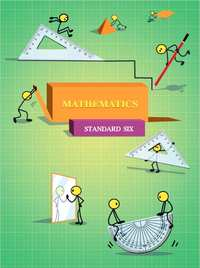## Chapter 7: Symmetry

Practice Set 20Practice Set 21
Practice Set 20 [Page 41]

### Balbharati solutions for Mathematics 6th Standard Maharashtra State Board Chapter 7 Symmetry Practice Set 20 [Page 41]

Practice Set 20 | Q 1.1 | Page 41

Draw the axes of symmetry of the figure below. Which of them has more than one axis of symmetry?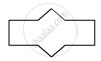Practice Set 20 | Q 1.2 | Page 41

Draw the axes of symmetry of the figure below. Which of them has more than one axis of symmetry?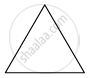Practice Set 20 | Q 1.3 | Page 41

Draw the axes of symmetry of the figure below. Which of them has more than one axis of symmetry?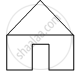Practice Set 20 | Q 1.4 | Page 41

Draw the axes of symmetry of the figure below. Which of them has more than one axis of symmetry?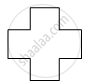Practice Set 20 | Q 2 | Page 41

Write the capital letters of the English alphabet in your notebook. Try to draw their axes of symmetry. Which ones have an axis of symmetry? Which ones have more than one axis of symmetry?

Practice Set 20 | Q 3 | Page 41

Use colour, a thread and a folded paper to draw symmetrical shapes.

Practice Set 20 | Q 4 | Page 41

Observe various commonly seen objects such as tree leaves, birds in flight, pictures of historical buildings, etc. Find symmetrical shapes among them and make a collection of them.

Practice Set 21 [Pages 42 - 21]

### Balbharati solutions for Mathematics 6th Standard Maharashtra State Board Chapter 7 Symmetry Practice Set 21 [Pages 42 - 21]

Practice Set 21 | Q 1.1 | Page 42

Along the figure shown below, a line l has been drawn. Complete the symmetrical figure by drawing a figure on the other side such that the line l becomes the line of symmetry.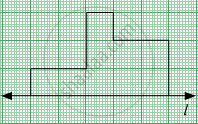Practice Set 21 | Q 1.2 | Page 42

Along the figure shown below, a line l has been drawn. Complete the symmetrical figure by drawing a figure on the other side such that the line l becomes the line of symmetry.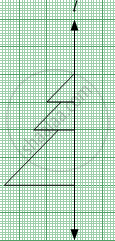Practice Set 21 | Q 1.3 | Page 21

Along the figure shown below, a line l has been drawn. Complete the symmetrical figure by drawing a figure on the other side such that the line l becomes the line of symmetry.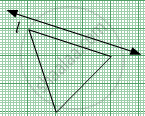## Chapter 7: Symmetry

Practice Set 20Practice Set 21## Balbharati solutions for Mathematics 6th Standard Maharashtra State Board chapter 7 - Symmetry

Balbharati solutions for Mathematics 6th Standard Maharashtra State Board chapter 7 (Symmetry) include all questions with solution and detail explanation. This will clear students doubts about any question and improve application skills while preparing for board exams. The detailed, step-by-step solutions will help you understand the concepts better and clear your confusions, if any. Shaalaa.com has the Maharashtra State Board Mathematics 6th Standard Maharashtra State Board solutions in a manner that help students grasp basic concepts better and faster.

Further, we at Shaalaa.com provide such solutions so that students can prepare for written exams. Balbharati textbook solutions can be a core help for self-study and acts as a perfect self-help guidance for students.

Concepts covered in Mathematics 6th Standard Maharashtra State Board chapter 7 Symmetry are Concept of Symmetry, Concept of Reflection Symmetry, Drawing a Symmetric Figure on a Graph.

Using Balbharati 6th Standard solutions Symmetry exercise by students are an easy way to prepare for the exams, as they involve solutions arranged chapter-wise also page wise. The questions involved in Balbharati Solutions are important questions that can be asked in the final exam. Maximum students of Maharashtra State Board 6th Standard prefer Balbharati Textbook Solutions to score more in exam.

Get the free view of chapter 7 Symmetry 6th Standard extra questions for Mathematics 6th Standard Maharashtra State Board and can use Shaalaa.com to keep it handy for your exam preparation